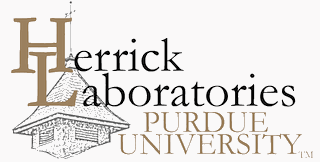## International Compressor Engineering Conference

1171

2012

#### Keywords

Compression volume, Geometry simulation, Greene formula, interesting contour, Port area, Compression process simulation

#### Abstract

Thermodynamic models to simulate positive displacement compressors are well known for a few decades already and can describe the compressor performance quite well. One of the most complex part of the simulation process however is the positive displacement compressor geometry simulation. In the current context, under geometry simulation, values of working compressor volumes, as well as cross-sections of port openings, at different shaft (rotor) angles are considered.In the current paper, as an example, the Greene formula approach was applied to a geometry simulation of a single screw compressor. Working chamber geometry was defined by “cutting “ it with the gate rotor blade; flute volume was computed using Greene formula; suction and discharge ports were intersected with this volume so that port opening contours were defined and their respective cross sectional areas were calculated. The results of this geometry simulation were applied for a thermodynamic model of a single screw compressor. Experimental investigation of a single screw working process using pressure transducers has been conducted. Geometry simulation data was applied to calculate compression work from experimental pressure traces.Pressure-volume diagrams obtained experimentally were compared to the simulated ones. Also, indicator work obtained from simulation and experiment, was compared to the power input to the shaft, and demonstrated good correlation.

COinS